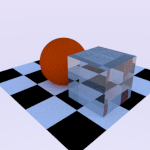# Index of refractionBy introducing transparent materials We have seen different parameters that characterize. In “Cycles” have named a shader “Glass” to simulate the behavior of translucent objects where the light changes direction through them.

Has an associated transparency parameter that redirection of light rays passing through the material, is the coefficient of refraction; the software performs calculations to simulate these effects are based on the "Ley de Snell".

Refraction is the change of direction experienced a wave when passing from one material medium to another. Only occurs if the obliquely incident wave on the interface of two media, and if they have different refractive indices. Originates refraction change speed of wave propagation. (Wikipedia)Law of refraction (Ley de Snell) (Source wikipedia)

This law tells us how changes direction a ray of light passing from one medium to another, for example from water to air, by impinging under a certain angle with respect to the normal to the surface separating two media, and is modeled according to the following expression:Where:

• n1 = refractive index of the first medium,
• θ1 = Angle of Incidence,
• n2 = refractive index as it means
• θ2 = angle of refraction.

The result is that objects that lie behind the transparent object are displaced by changing the light reflected from them your address. To see this graphically physical effect we have prepared a few images that we will increase the refractive index. We see the back image shift (sphere) on the faces of the cube.

In the pictures below we will increase the IOR (“Index Of Refraction”)

Finally we can see the following animated gif in which we vary the refractive index continuously to see its effect. An interesting example.